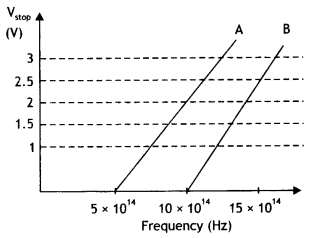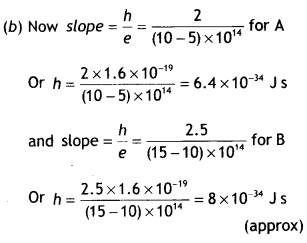Here we are providing Class 12 Physics Important Extra Questions and Answers Chapter 11 Dual Nature of Radiation and Matter. Important Questions for Class 12 Physics with Answers are the best resource for students which helps in Class 12 board exams.

## Class 12 Physics Chapter 11 Important Extra Questions Dual Nature of Radiation and Matter

### Dual Nature of Radiation and Matter Important Extra Questions Very Short Answer Type

Question 1.
If the maximum kinetic energy of electrons emitted by photocell is 4 eV, what is the stopping potential?
The stopping potential is 4 V.

Question 2.
Two metals A and B have a work function 4 eV and 10 eV respectively. Which metal has a higher threshold wavelength?
The threshold wavelength is inversely proportional to the work function. Therefore metal A has a higher threshold wavelength.

Question 3.
Ultraviolet light is incident on two photo-sensitive materials having work functions W1 and W2 (W1 > W2). In which case will the kinetic energy of the emitted electrons be greater? Why?
The kinetic energy of the emitted photoelectrons is given by 1/2 mv² = hv – W; therefore the lesser the work function for a given frequency, the more is the kinetic energy of the emitted photoelectrons. Since W2 < W1, kinetic energy will be more for the metal having work function W2.

Question 4.
Does the threshold frequency depend on the intensity of light?
No, it does not.

Question 5.
Name the experiment which establishes the wave nature of a particle.
Davison-Germer experiment.

Question 6.
Mention one physical process for the release of electrons from a metal surface.
Photoelectric effect.

Question 7.
Name a phenomenon that illustrates the particle nature of light.
Photoelectric effect.

Question 8.
Define the work function for a given metallic surface.
The minimum amount of energy required to just eject an electron from a given metal surface is called the work function for that metal surface.

Question 9.
What is the value of stopping potential between the cathode and anode of a photocell, if the maximum kinetic energy of the electrons emitted is 5 eV?
Stopping potential = 5 V.

Question 10.
Define the term ‘stopping potential’ in relation to the photoelectric effect. (CBSE AI 2011)
It is the negative potential of the collector plate for which no photoelectron reaches the collector plate.

Question 11.
Write the relationship of de-Broglie wavelength associated with a particle of mass m in terms of its kinetic energy E. (CBSE Delhi 2011C)
The required relation is λ = $$\frac{h}{\sqrt{2 m E}}$$

Question 12.
State de-Broglie hypothesis. (CBSE Delhi 2012)
It states that moving particles should possess a wave nature.

Question 13.
Define the term “threshold frequency”, in the context of photoelectric emission. (CBSE Delhi 2019)
It is the minimum frequency of incident radiation (light) that can cause photoemission from a given photosensitive surface.
Or
Define the term “Intensity” in the photon picture of electromagnetic radiation. (CBSE Delhi 2019)
It is defined as the number of energy quanta (photons) per unit area per unit time.

Question 14.
Write the expression for the de-Broglie wavelength associated with a charged particle having charge ‘q’ and mass ‘m’, when it is accelerated by a potential V. (CBSE AI 2013)
The expression is λ = $$\frac{h}{\sqrt{2 m q V}}$$

Question 15.
Define the intensity of radiation on the basis of the photon picture of light. Write its SI unit. (CBSE AI 2014)
The intensity of radiation is defined as the number of energy quanta per unit area per unit time. It is measured in W m-2.

Question 16.
Name the phenomenon which shows the quantum nature of electromagnetic radiation. (CBSE Al 2017)
Photoelectric effect.

Question 17.
If the distance between the source of light and the cathode of a photocell is doubled how does it affect the stopping potential applied to the photocell? (CBSE Delhi 2017C)
No effect.

Question 18.
Draw graphs showing the variation of photoelectric current with applied voltage for two incident radiations of equal frequency and different intensities. Mark the graph for the radiation of higher intensity. (CBSE AI 2018)
The graphs are as shown.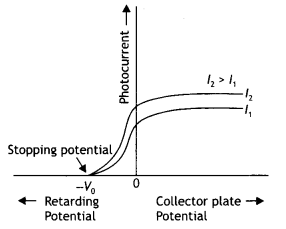Question 19.
An electron is accelerated through a potential difference V. Write the expression for its final speed if it was initially at rest. (CBSE AI Delhi 2018C)
Using eV = $$\frac{1}{2}$$mv² or v = $$\sqrt{\frac{2 e V}{m}}$$

Question 20.
Write the name given to the frequency v0, in the following graph (showing the variation of stopping potential (Vo) with the frequency (v) of the incident radiation) for a given photosensitive material. Also name the constant, for that photosensitive material, obtained by multiplying vc with Planck’s constant.The constant obtained by multiplying v0 with Planck’s constant is called the work function of the material. This frequency is called the threshold frequency.

Question 21.
Electrons are emitted from a photosensitive surface when it is illuminated by green light but electron emission does not take place by yellow light. Will the electrons are emitted when the surface is illuminated by
(a) red light
No electron will be emitted when illuminated by a red light.

(b) blue light?
Electron emission takes place with blue light.

Question 22.
The frequency (v) of incident radiation is greater than the threshold frequency v0 in a photocell. How will the stopping potential vary if frequency (v) is increased keeping other factors constant?
On increasing the frequency v of the incident light, the value of stopping potential also increases.

Question 23.
How much time is taken by a photoelectron to come out of a metal surface, when the light of wavelength less than threshold wavelength λo is incident on it?
It is an instantaneous process. The time required is of the order of a nanosecond (10-9 s).

Question 24.
The given graphs show the variation of photoelectric current (l) with the applied voltage (V) for two different materials and for two different intensities of the incident radiations. Identify the pairs of curves that correspond to different materials but the same intensity of incident radiations.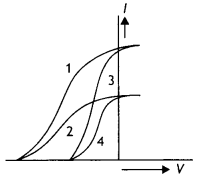Pairs 1 – 2 and pairs 3 – 4

Question 25.
Red light, however bright, cannot cause the emission of electrons from a clean zinc surface. But even weak ultraviolet radiations can do so. Why?
This is because the threshold frequency of the given metal is greater than the frequency of red light.

Question 26.
If the intensity of incident radiation on metal is doubled, what happens to the kinetic energy of electrons emitted?
The kinetic energy of emitted electrons remains unchanged.

Question 27.
If the intensity of the incident radiation in a photocell is increased, how does the stopping potential vary?
Stopping potential remains unaffected.

Question 28.
The work function of aluminium is 4.2 eV. If two photons each of energy 2.5 eV are incident on a surface, will the emission of photoelectron take place?
No, emission of photoelectron will not take place.

Question 29.
A photon and an electron have the same de-Broglie wavelength. Which is moving faster?
Photon is moving faster with a speed c = 3 × 108 ms-1. An electron must have a velocity less than the speed of light.

Question 30.
Does the ‘stopping potential’ in photoelectric emission depend upon
(i) the intensity of the incident radiation in a photocell
No

(ii) the frequency of the incident radiation?
Yes.

Question 31.
The de-Broglie wavelengths, associated with a proton and a neutron, are found to be equal. Which of the two has a higher value for kinetic energy?
Proton.

Question 32.
The figure shows a plot of $$\frac{1}{\sqrt{V}}$$ where V is the accelerating potential vs the de-Broglie wavelength λ in case of two particles having the same charge q but different masses m1 and m2. Which line A or B represents the particle of greater mass? (CBSE AI 2013C)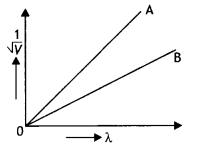Particle A

Question 33.
Do X-rays exhibit the phenomenon of the photoelectric effect?
Yes, they do exhibit the phenomenon of the photoelectric effect.

Question 34.
Draw a graph showing the variation of de-Broglie wavelength with the momentum of an electron. (CBSE AI 2019)
The graph is shown below.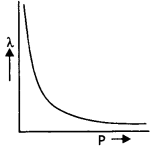Question 35.
The work function of two metals A and B are 2 eV and 5 eV respectively. Which of these is suitable for a photoelectric cell using visible tight?
Metal A having a lower work function of 2 eV is suitable for use with visible light.

Question 36.
Estimate the frequency associated with a Photon of energy 2 eV. (CBSE Delhi 2019C)
Since E = hv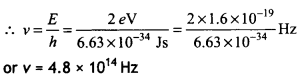Question 37.
For a given photosensitive material and with a source of the constant frequency of incident radiation, how does the photocurrent vary with the intensity of incident light? (CBSE AI 2011C)
The photoelectric current increases linearly with the intensity of light.

Question 38.
Draw a graph showing the variation of the de-Broglie wavelength of an electron as a function of its kinetic energy. (CBSE Delhi 2015C)
The graph is as shown.Question 39.
(a) In the explanation of the photoelectric effect, we assume one photon of frequency v collides with an electron and transfers its energy. This leads to the equation for the maximum energy Emax of the emitted electron as Emax = hv – Φo where Φo is the work function of the metal. If an electron absorbs 2 photons (each of frequency v), what will be the maximum energy for the emitted electron?
Emax = 2hv – Φ

(b) Why is this fact (two photons absorption) not taken into consideration in our discussion of the stopping potential? (NCERT Exemplar)
The probability of absorbing 2 photons by the same electron is very low. Hence ’ such emissions will be negligible.

Question 40.
Do all the electrons that absorb a photon comes out as photoelectrons? (NCERT Exemplar)
No, most electrons get scattered into the metal. Only a few come out of the surface of the metal.

### Dual Nature of Radiation and Matter Important Extra Questions Short Answer Type

Question 1.
An a-particle and a proton of the same kinetic energy are in turn allowed to pass through a magnetic field B, acting normal to the direction of motion of the particles. Calculate the ratio of radii of the circular paths described by them. (CBSE Delhi 2019)
Given qα = 2e, qp = e, Kα = Kp, mα = 4mp, rα/rp = ?
Using the expression
r = $$\frac{\sqrt{2 m K}}{q B}$$ we haveQuestion 2.
How will the photoelectric current change on decreasing the wavelength of incident radiation for a given photosensitive material?
Photoelectric current is independent of the wavelength of the incident radiation. Therefore there will be no change in the photoelectric current.

Question 3.
Estimate the ratio of the wavelengths associated with the electron orbiting around the nucleus in the ground and first excited states of a hydrogen atom. (CBSE Delhi 2019C)
Since De Brogue’s hypothesis is related to
Bohr’s atomic model as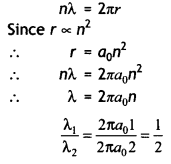Question 4.
Show graphically how the stopping potential for a given photosensitive surface varies with the frequency of the incident radiation.
The required graph is as shown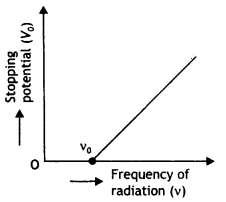Question 5.
the de-Broglie wavelength associated with an electron accelerated through a potential difference V is λ. What will be its wavelength when accelerating potential is increased to 4 V?
The de-BrogLie wavelength is inversely proportional to the square root of potential, therefore = $$\frac{\lambda_{2}}{\lambda_{1}}=\frac{\sqrt{V}}{\sqrt{4 V}}=\frac{1}{2}$$ . Thus wavelength
wilt become half of its previous value.

Question 6.
Plot a graph showing the variation of de Brogue wavelength (λ) associated with a charged particle of mass m, versus $$\frac{1}{\sqrt{V}}$$ where V is the potential difference through which the particle is accelerated. How does this graph give us information regarding the magnitude of the charge of the particle? (CBSE Dethi 2019)
The plot is as shown.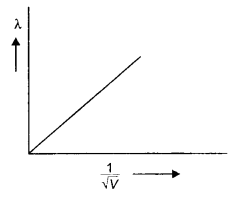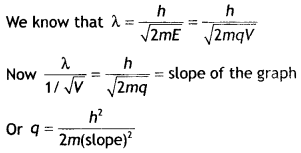Question 7.
X-rays of wavelength ‘λ’ fall on a photosensitive surface, emitting electrons. Assuming that the work function of the surface can be neglected, prove that the de-Broghe wavelength of the electrons emitted will be $$\sqrt{\frac{h \lambda}{2 m c}}$$
The energy possessed by X-rays of wavelength λ is given by E=hc / λ.
Consider an electron of mass charge e to be accelerated the potential difference of V volts the velocity gained by it.

Then kinetic energy of electron is
E = $$\frac{1}{2} m v^{2}$$ = eV
or
v = $$\sqrt{\frac{2 \mathrm{eV}}{\mathrm{m}}}=\sqrt{\frac{2 E}{m}}$$

If λ is the de-Broglie wavelength associated with an electron, thenSubstituting for e, we have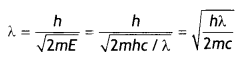Question 8.
Explain with the help of Einstein’s photoelectric equation any two observed features in the photoelectric effect. cannot be explained by the wave theory. (CBSE Delhi 2019)
According to Einstein’s equation, we have
$$\frac{1}{2} m v_{\max }^{2}$$ = h(v – vo)

Two features
(a) Maximum energy is directly proportional to the frequency
(b) Existence of threshold frequency Explanation of two features:

1. The energy of the photon is directly proportional to the frequency
2. No photoelectric emission is possible if hv < hvo

Question 9.
Why is the wave theory of electromagnetic radiation not able to explain the photoelectric effect? How does the photon picture resolve this problem? (CBSE Delhi 2019)
According to the wave theory, the more intense a beam, more is the kinetic energy it will impart to the photoelectron. This does not agree with the experimental observations (max K.E. of the emitted photoelectron is independent of intensity) on the photoelectric effect. Also according to the wave theory photoemission can occur at all frequencies.

The photon picture resolves this problem by saying that light in interaction with matter behaves as if it is made of quanta or packets of energy, each of energy hv. This picture enables us to get a correct explanation of all the observed experimental features of the photoelectric effect.

Question 10.
(a) Define the terms,
(i) threshold frequency and
(ii) stopping potential in the photoelectric effect.
(b) Plot a graph of photocurrent versus anode potential for radiation of frequency v and intensities l1 and l2. (l1 < l2). (CBSE Delhi 2019)
(a) Threshold frequency: It is the frequency of the incident radiation below which photoelectric effect does not take place.
Stopping potential: It is the minimum negative (retarding) potential, given to the anode (collector plate) for which the photocurrent stops or becomes zero.

(b) The plot is as shown.Question 11.
A proton and a particle are accelerated through the same potential difference. Which one of the two has
(i) greater de-Broglie wavelength, and
(i) We know that λ = $$\frac{h}{\sqrt{2 m q V}}$$, therefore
we have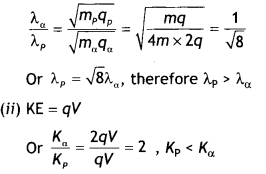Question 12.
Plot a graph showing the variation of de-Broglie wavelength λ versus $$\frac{1}{\sqrt{V}}$$ where V is the accelerating potential for two particles A and B carrying the same charge but masses m1 m2 (m1 > m2). Which one of the two represents a particle of smaller mass and why? (CBSE Delhi 2016)
The graph is as shown.we know that λ = $$\frac{h}{\sqrt{2 m q V}}$$

In graphs, charge of both particles is the same, but the slope of graph B is more. It means that mass of particle B is less (since slope ∝ $$\frac{1}{\sqrt{m}}$$

Question 13.
For a photosensitive surface, the threshold wavelength is λo. Does photoemission occur if the wavelength λ of the incident radiation is
(i) more than λo and
The photoelectric effect occurs and hence photoelectrons are ejected when the wavelength of the incident radiation is lesser than the threshold wavelength.
(i) When λ > λo, photoemission does not take place.
(ii) When λ < λo, photoemission takes place.

Question 14.
Two monochromatic radiations of frequencies v1 and v2 (v1 > v2) and having the same intensity is, in turn, an incident on a photosensitive surface to cause photoelectric emission. Explain, giving a reason, in which case (i) more number of electrons will be emitted and (ii) maximum kinetic energy of the emitted photoelectrons will be more. (CBSE Delhi 2014C)
The number of photoelectrons emitted depends upon the intensity of radiation and the kinetic energy of photoelectrons depends upon the frequency of radiation, therefore

1. The same number of electrons will be emitted.
2. Photoelectrons will have more kinetic energy for radiation of frequency v1.

Question 15.
A proton and an a-particle are accelerated, using the same potential difference. How are the de-Broglie wavelengths λP and λα related to each other? (NCERT Exemplar)Question 16.
There are materials that absorb photons of shorter wavelength and emit photons of longer wavelength. Can there be stable substances that absorb photons of larger wavelength and emit light of shorter wavelength? (NCERT Exemplar)
In the first case energy given out is less than the energy supplied. In the second case, the material has to supply energy as the emitted photon has more energy. This cannot happen for stable substances.

Question 17.
Two monochromatic beams A and B of equal intensity l hit a screen. The number of photons hitting the screen by beam A is twice that by beam B. Then what inference can you make about their frequencies? (NCERT Exemplar)The frequency of beam B is twice that of beam A.

Question 18.
Two particles A and B of de Broglie wavelengths λ1 and λ2 combine to form a particle C. The process conserves momentum. Find the de Broglie wavelength of the particle C. (The motion is one dimensional.) (NCERJExemplar)
The motion is one dimension, therefore
(i) If the particles move in the same direction(ii) If the particles move in the opposite directionQuestion 19.
Find the frequency of light that ejects electrons from a metal surface, fully stopped by a retarding potential of 3.3 V. If photoelectric emission begins in this metal at a frequency of 8 × 1014 Hz, calculate the work function (in eV) for this metal.
Or
Monochromatic light of frequency 6.0 × 1014 Hz is produced by a laser. The power emitted is 2.0 × 10-3W. Calculate the (i) energy of a photon in the light beam and (ii) the number of photons emitted on an average by the source. (CBSE Delhi 2018C)
We have### Dual Nature of Radiation and Matter Important Extra Questions Long Answer Type

Question 1.
What is the photoelectric effect? Write Einstein’s photoelectric equation and use it to explain (a) independence of maximum energy of emitted photoelectrons from the intensity of incident light and
(b) existence of a threshold frequency for the emission of photoelectrons.
The election of photoelectrons from a metal surface when Light of suitabLe frequency is incident on it is catted photoelectric effect.

Einstein’s equation of photoelectric effect is $$\frac{1}{2}$$mv² = hv – ω0
(a) In accordance with Einstein’s equation, the kinetic energy of the photoelectrons is independent of the intensity of the incident radiation.
(b) In accordance with Einstein’s equation, the kinetic energy will be positive and hence photoelectrons will be ejected if v > v0. Thus below a certain frequency called threshold frequency, photoelectrons are not ejected from a metal surface (if v < v0).

Question 2.
An electron of mass m and charge q is accelerated from rest through a potential difference of V. Obtain the expression for the de-Broglie wavelength associated with it. If electrons and protons are moving with the same kinetic energy, which one of them will have a larger de-Broglie wavelength associated with it? Give reason.
Consider an electron of mass m and charge e to be accelerated through a potential difference of V volts. Let v be the velocity gained by it. Then kinetic energy of the electron is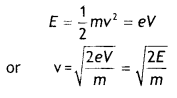If λ is the de-Broglie wavelength associated with an electron, then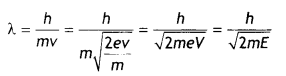Since de-Broglie wavelength is inversely proportional to the square root of mass, the lesser the mass, the more is the de- Broglie wavelength. Since the mass of an electron is lesser than that of the proton, the electron has a greater de-Broglie wavelength than a proton.

Question 3.
Sketch the graphs showing the variation of stopping potential with the frequency of incident radiations for two photosensitive materials A and B having threshold frequencies v0 > v’0 respectively.
(a) Which of the two metals A or B has a higher work function?
(b) What information do you get from the slope of the graphs?
(c) What does the value of the intercept of graph ‘A’ on the potential axis represent?
The graphs are as shown below.(a) The work function is directly proportional to the threshold frequency. The threshold frequency of metal A is greater than that of metal B; therefore A has a greater work function than B.
(b) The slope of the graphs gives the value of Planck’s constant.
(c) The intercept on the potential axis is negative (-W0/e) w.r.t. stopping potential, i.e. Work function = e × magnitude of the intercept on the potential axis. We may infer it to give the voltage which, when applied with opposite polarity to the stopping voltage, will just pull out electrons from the metallic atom’s outermost orbit.

Question 4.
When a given photosensitive material is irradiated with light of frequency v, the maximum speed of the emitted photoelectrons equals Vmax. The graph shown in the figure gives a plot of V²max varying with frequency v.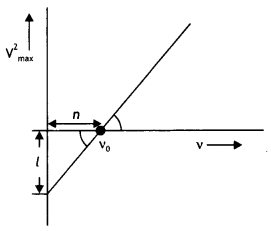Obtain an expression for
(a) Planck’s constant, and
By Einstein’s photoelectric equation we have(b) The work function of the given photosensitive material in terms of the parameters T, ‘n’ and the mass ‘m’ of the electron.
The intercept on V²max axis is = $$\frac{2 \phi_{o}}{m}$$ = l
Therefore, work function Φ0 = $$\frac{ml}{2}$$

(c) How is threshold frequency determined from the plot? (CBSE AI 2019)
The threshold frequency is the intercept on the v axis i.e. v0 = n

Question 5.
X-rays fall on a photosensitive surface to cause photoelectric emission. Assuming that the work function of the surface can be neglected, find the relation between the de-Broglie wavelength (λ) of the electrons emitted to the energy (Ev) of the incident photons. Draw the nature of the graph for λ as a function of Ev. (CBSE Delhi 2014C)
Consider an electron of mass m and charge e to be accelerated through a potential difference of V volt. Let v be the velocity gained by it. Then kinetic energy of the electron is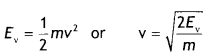If λ is the de-Broglie wavelength associated with an electron, then
λ = $$\frac{h}{m v}=\frac{h}{\sqrt{2 m E_{v}}}$$

The nature of the graph is as shown.Question 6.
Light of intensity ‘l’ and frequency ‘v’ is incident on a photosensitive surface and causes photoelectric emission. What will be the effect on anode current when
(a) the intensity of light is gradually increased,
(b) the frequency of incident radiation is increased and
(c) the anode potential is increased?
In each case, all other factors remain the same. Explain giving justification in each case. (CBSE AI 2015)
(a) Anode current will increase with the increase of intensity as the more the intensity of light, the more is the number of photons and hence more number of photoelectrons are ejected.
(b) No effect as the frequency of light affects the maximum K.E. of the emitted photoelectrons.
(c) Anode current will increase with anode potential as more anode potential will accelerate the more electrons till it attains a saturation value and gets them collected at the anode at a faster rate.

Question 7.
The graphs, drawn here, are for the phenomenon of the photoelectric effect.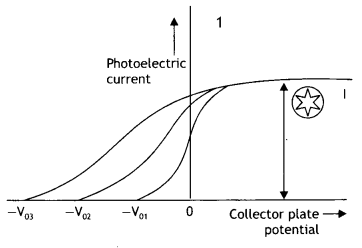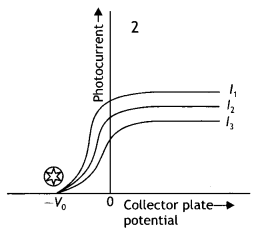(a) Identify which of the two characteristics (intensity/frequency) of incident light is being kept constant in each case.
Graph 1: Intensity, Graph 2: Frequency

(b) Name the quantity, corresponding to the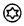mark, in each case.
Graph 1: Saturation current, Graph 2: stopping potential

(c) Justify the existence of a ‘threshold frequency’ for a given photosensitive surface. (CBSE Delhi 2016C)
The electrons require minimum energy to set themselves free. This is called the work function. As the energy of the photon depends upon its frequency, the photons must possess a minimum frequency so that their energy becomes equal to or greater than the work function. This is called threshold frequency and is given by v0 = $$\frac{\omega_{0}}{h}$$

Question 8.
Draw a graph showing the variation of de-Broglie wavelength λ of a particle of charge q and mass, with the accelerating potential V. An alpha particle and a proton have the same de-Broglie wavelength equal to 1 Å. Explain with calculations, which of the two has more kinetic energy. (CBSE Delhi 2017C)
The graph is as shown.
The de-Broglie wavelength of a particle is given by the expression λ = $$\frac{h}{\sqrt{2 m q V}}$$

Since the alpha particle and the proton have the same de- Broglie wavelength, we have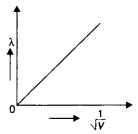$$\frac{h}{\sqrt{2 m_{a} E_{\alpha}}}=\frac{h}{\sqrt{2 m_{p} E_{p}}}$$

Therefore proton has a greater value of de-Broglie wavelength.
Now kinetic energy is given by the expression
$$\frac{E_{a}}{E_{p}}=\frac{m_{p}}{m_{\alpha}}=\frac{m}{4 m}=\frac{1}{4}$$

Thus proton has more kinetic energy.

Question 9.
(a) Plot a graph showing the variation of photoelectric current with collector plate potential for different frequencies but of the same intensity of incident radiation.
The graph between current I and plate potential.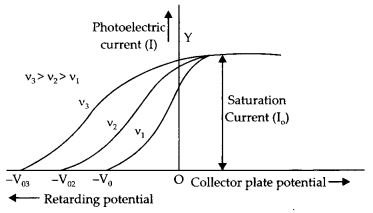(b) Green and blue light of the same intensity are incident separately on the same photosensitive surface. If both of these cause photoelectric emission, which one will emit photoelectrons
(i) having greater kinetic energy and
The blue light will emit photoelectrons having greater kinetic energy because the frequency and hence energy (E = hv) of blue light is more than the frequency and hence the energy of green light.

Photoelectric current will be nearly the same for the blue and green light because the intensity of saturation current is independent of the frequency of incident light.

Question 10.
Radiation of frequency 1015 Hz is incident on two photosensitive surfaces P and Q. The following observations were recorded:
(a) Surface P: No photoemission occurs.
(b) Surface Q: Photoemission occurs but photoelectrons have zero kinetic energy. Based on Einstein’s photoelectric equation, explain the two observations.
Einstein photoelectric equation is h(v – v0) = $$\frac{1}{2}$$ mv²
(a) As in the case of surface P no photoemission takes place, we conclude that threshold frequency for P has a value greater than the frequency 1015 Hz of the given radiation.
(b) In the case of surface Q photoemission takes place but the kinetic energy of photoelectrons means that this radiation just overcomes the work function of the metal. In other words, the frequency 1015 Hz, of this radiation is equal to the threshold frequency.

Question 11.
When the light of frequency v1 is incident on a photosensitive surface, the stopping potential is V1 If the frequency of incident radiation becomes v1/2, the stopping potential changes to V2. Find out the expression for the threshold frequency for the surface in terms of V1 and V2.
If the frequency of incident radiation is doubled, will the maximum kinetic energy of the photoelectrons also be doubled? Give reason. (CBSE AI 2019)
By Einstein’s photoelectric equation.
eV1 = hv1 – hv0 …(i).
and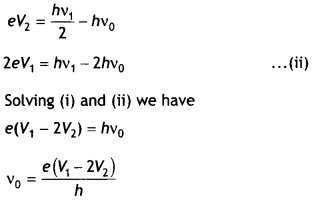If the frequency is doubled, the maximum kinetic energy will not be doubled.
Kmax = hv – hv0
Knew = 2hv – hv0 = hv + Kmax

Question 12.
(a) Define the terms
(i) threshold frequency and
Threshold frequency (v0) is the minimum value of frequency of incident radiations below which the photoelectric emission stops, altogether.

(ii) stopping potential in the context of the photoelectric effect.
Stopping potential is the value of retarding potential at which the photoelectric current becomes zero for the given frequency of incident radiations.

(b) Draw a graph showing the variation of stopping potential (V0) with frequency (v) of incident radiation for a given photosensitive material. (CBSE 2019C)
The graph between v and V0Question 13.
State Einsteins photoelectric equation explaining the symbols used.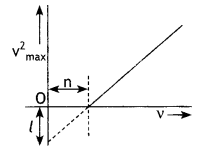Light of frequency y is incident on a photosensitive surface. A graph of the square of the maximum speed of the electrons (v2max) vs. y is obtained as shown in the figure. Using Einstein’s photoelectric equation, obtain expressions for (i) Planck’s constant and (ii) the work function of the given photosensitive material in terms of parameters l, n and mass of the electron m. (CBSE Delhi 2018C)
Einstein’s photoelectric equation is
hv = hv0 (= ω0) = $$\frac{1}{2}$$mv2max
v = frequency of incident Light
ω0 = threshold frequency of photosensitive A material.
ω0 = work function.
$$\frac{1}{2}$$mv2max = max. the kinetic energy of the emitted photoelectrons

(Also accept if the student writes)
hv = ω0 + eVs
ω0 = Work function of photosensitive material
Vs = stopping potential)

From Einstein’s photoelectronic equation,
we have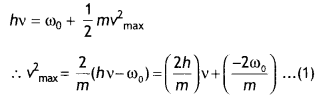(i) Slope of the given graph = $$\frac{l}{n}$$
∴ $$\frac{2 h}{m}=\frac{l}{n}$$
or
h = $$\frac{m l}{2 n}$$

(ii) Intercept on the y-axis = – l
From equation (1)
– l = $$\frac{-2 \omega_{0}}{m}$$ ⇒ ω0 = $$\frac{ml}{2}$$

Question 14.
(a) In the photoelectric effect, do all the electrons that absorb a photon comes out as photoelectrons irrespective of their location? Explain.
No, it is not necessary that if the energy supplied to an electron is more than the work function, it will come out. The electron after receiving energy may lose energy to the metal due to collisions with the atoms of the metal. Therefore, most electrons get scattered into the metal. Only a few electrons near the surface may come out of the surface of the metal for whom the incident energy is greater than the work function of the metal.

(b) A source of light, of frequency greater than the threshold frequency, is placed at a distance be ‘d’ from the cathode of a photocell. The stopping potential is found to be V. If the distance of the light source is reduced to d/n (where n > 1), explains the changes that are likely to be observed in the (a) photoelectric current and (b) stopping potential. (CBSE Sample Paper 2018-19)

1. On reducing the distance, intensity increases. Photoelectric current increases with the increase in intensity.
2. Stopping potential is independent of intensity and therefore remains unchanged.

Question 15.
Explain the laws of the photoelectric effect on the basis of Einstein’s photoelectric equation.
The laws of the photoelectric effect can be explained on the basis of Einstein’s photoelectric equation, i.e.
$$\frac{1}{2}$$mv² = h (v – v0)
(a) From the equation we find that the kinetic energy will be positive and hence photoelectrons will be emitted if v > v0, i.e. the incident frequency is greater than the threshold frequency.

(b) It is clear from the expression that the kinetic energy of the emitted photoelectrons varies linearly with the frequency of the incident radiation.

(c) According to Einstein highly energetic radiation has a high frequency and an intense beam of radiation has more photons. Therefore when a high-intensity beam of radiation is incident on the metal surface, a large number of photoelectrons are emitted as the photoelectric effect is a one-one phenomenon, i.e. one photon can eject one photoelectron. Thus the more the intensity of the incident radiation, the more is the photoelectric current.

(d) As soon as a photon is an incident on a metal surface it is immediately absorbed by an electron, which sets itself free and comes out of the metal surface. The time lag is being negligible. Thus the photoelectric effect is instantaneous.

Question 16.
Define the terms threshold frequency and stopping potential in relation to the phenomenon of the photoelectric effect. How is the photoelectric current affected on increasing the
(i) frequency and
(ii) the intensity of the incident radiations and why?
Stopping potential (V0): The negative potential of the plate at which no photoelectrons reach is called the stopping potential or the cut-off potential.

Threshold frequency (v0): The minimum frequency of the incident radiation, which can eject photoelectrons from a material, is known as the threshold frequency or cut-off frequency of the material.

1. When the frequency has increased the energy absorbed by a single electron on collision with a photon also increases. E= hv, h is Planck’s constant. This raises the kinetic energy of the emitted photoelectrons but has no effect on current as the number of electrons being liberated remains the same.
2. When the intensity of the incident radiation is increased, the number of photons crossing a unit area per second increases. These photons liberate more electrons and hence current increases.

Question 17.
Write Einstein’s photoelectric equation and mention which important features in the photoelectric effect can be explained with the help of this equation.
The maximum kinetic energy of the photoelectrons gets doubled when the wavelength of light incident on the surface changes from λ1 to λ2. Derive the expressions for the threshold wavelength λ0 and work function for the metal surface. (CBSE Delhi 2015)
Einstein’s equation is
Emax = hv – ω or $$\frac{1}{2}$$mv²max = hv – ω

Here m is the mass of the photoelectron and vmax is maximum velocity.
(a) In accordance with Einstein’s equation, the kinetic energy of the photoelectrons is independent of the intensity of the incident radiation.
(b) In accordance with Einstein’s equation, the kinetic energy will be positive and hence photoelectrons will be ejected if v > vo. Thus below a certain frequency called the threshold frequency, photoelectrons are not ejected from a metal surface.
Given E1 = E, E2 = 2E,
By Einstein’s equation we haveQuestion 18.
Point out two distinct features observed experimentally in the photoelectric effect which cannot be explained on the basis of the wave theory of light. State how the ‘photon picture’ of light provides an explanation of these features. (CBSE AI 2016C)
The two features are

1. The intensity of light determines the photoelectric current and
2. Existence of threshold frequency.

According to the wave picture of light, the free electrons at the surface of the metal (over which the beam of radiation falls) absorb the radiant energy continuously. The greater the intensity of radiation, the greater is the amplitude of electric and magnetic fields. Consequently, the greater the intensity, the greater should be the energy absorbed by each electron. In this picture, the maximum kinetic energy of the photoelectrons on the surface is then expected to increase with the increase in intensity.

Also, no matter what the frequency of radiation is, a sufficiently intense beam of radiation (over sufficient time) should be able to impart enough energy to the electrons so that they exceed the minimum energy needed to escape from the metal surface. A threshold frequency, therefore, should not exist, which is against the observations. Thus the photoelectric effect cannot be explained on the basis of the wave nature of light

Question 19.
Using photon picture of light, show how Einstein’s photoelectric equation can be established. Write two features of the photoelectric effect that cannot be explained by the wave theory. (CBSE AI 2017)
According to Einstein, the emission of a photoelectron is the interaction of quanta of light with a single electron. The photon is completely absorbed by the electron. The electron, which absorbs the photon, utilises this energy to set itself free. An amount of energy equal to the work function (ω) is required by the electron to set itself free. The remaining energy hv – ω (if hv > ω) is available to the electron as its maximum kinetic energy. Thus according to Einstein, we have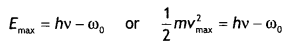Here m is the mass of the photoelectron and vmax is maximum velocity. Actually, most of the electrons possess kinetic energy less than the maximum kinetic energy because they lose a part of their energy due to collisions with the atoms on their way out from inside the metal. Thus electrons with different kinetic energy are emitted.

Now the work function is given by ω = hvo, therefore the above equation becomes
$$\frac{1}{2}$$mv²max = hv – hvo = h(v – vo)

(a) Dependence of kinetic energy on the frequency of the incident radiation.
(b) Existence of threshold frequency.

Question 20.
(a) How does one explain the emission of electrons from a photosensitive surface with the help of Einstein’s photoelectric equation?
(b) The work function of the following metals is given: Na = 2.75 eV, K = 2.3 eV, Mo = 4.17eV and Ni = 5.15 eV. Which of these metals will not cause photoelectric emission for radiation of wavelength 3300 Å from a laser source placed 1 m away from these metals? What happens if the laser source is brought nearer and placed 50 cm away? (CBSE Delhi 2017)
(a) According to Einstein’s equation we have
$$\frac{1}{2}$$mv² = h (v – vo)

If the frequency of the incident radiation is above the threshold frequency (vo), the KE of the photo¬electrons will be positive and hence will be emitted.

Let us calculate the energy possessed by the photon of wavelength 3300 Å.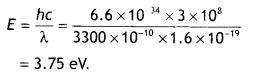As the energy of the incident photon is less than the work functions of Mo and Ni, so the metals Mo and Ni will not give photoelectric emission.

If the laser is brought closer, the intensity of radiation increases. This does not affect the result regarding Mo and Ni, but the photoelectric current will increase for Na and K with the increase in intensity.

Question 21.
When a monochromatic yellow coloured light beam is incident on a given photosensitive surface, photoelectrons are not ejected, while the same surface gives photoelectrons when exposed to the green coloured monochromatic beam. What will happen if the same surface is exposed to (i) violet and (ii) red coloured monochromatic beam of light? Justify your answer.
Photoelectrons are ejected from a metal surface if the frequency of incident radiation is greater than the threshold frequency of that metal.

Since yellow light does not eject photoelectrons while green light does, the threshold frequency of the given metal is greater than the frequency of yellow light.
(a) Violet light has a greater frequency than yellow light, therefore when violet light is incident on the metal surface photoelectrons will be ejected.
(b) Red light has a smaller frequency than yellow light; therefore when the red light is incident on the metal surface, photoelectrons will not be ejected.

Question 22.
(a) Why photoelectric effect cannot be explained on the basis of the wave nature of light? Give reasons.
According to the wave picture of light, the free electrons at the surface of the metal (over which the beam of radiation falls) absorb the radiant energy continuously. The greater the intensity of radiation, the greater is the amplitude of electric and magnetic fields.

Consequently, the greater the intensity, the greater should be the energy absorbed by each electron. In this theory, the maximum kinetic energy of the photoelectrons on the surface is then expected to increase with the increase in intensity.

Also, no matter what the frequency of radiation is, a sufficiently intense beam of radiation (over sufficient time) should be able to impart enough energy to the electrons so that they exceed the minimum energy needed to escape from the metal surface. A threshold frequency, therefore, should not exist, which is against the observations. Thus the photoelectric effect cannot be explained on the basis of the wave nature of light.

(b) Write the basic features of the photon picture of electromagnetic radiation on which Einstein’s photoelectric equation is based. (CBSE Delhi 2013)
The basic features are

1. Radiation energy is built up of discrete units.
2. An electron absorbs the quanta of energy.

Question 23.
Obtain the expression for the wavelength of the de-Broglie wave associated with an electron accelerated from rest through a potential difference V.
The two lines A and B as shown in the graph plot the de-Broglie wavelength A. as a function of 1/$$\sqrt{V}$$ (V is the accelerating potential) for two particles having the same charge. Which of the two represents the particle of heavier mass?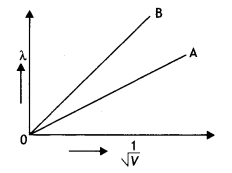Consider an electron of mass m and charge e to be accelerated through a potential difference of V volts. Let v be the velocity gained by it. Then kinetic energy of an electron is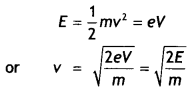If λ is the de-Broglie wavelength associated with an electron, thenIn graphs, the charge of both particLes is the same, but the slope of graph A is Less. It means that mass of particle A is more (since slope = $$\frac{h}{\sqrt{2 m E}}$$).

Question 24.
The given graphs show the variation of the stopping potential Vs with the frequency (v) of the incident radiations for two different photosensitive materials M1 and M2.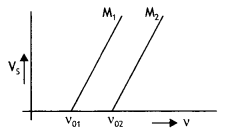(i) What are the values of work functions for M1 and M2?
(ii) The values of the stopping potential for M1 and M2 for a frequency (v3) of the incident radiations greater than vo2 are V1 and V2 respectively. Show that the slope of the lines equals $$\frac{V_{1}-V_{2}}{v_{02}-v_{01}}$$
(i) The values of work functions for M1 and M2 are ω1 = hvo1 and ω2 = hvo2.
(ii) The slope of the graphs is given by the variation of quantity along the Y-axis ‘ to the variation in quantity on the X-axis.

Now for M1Therefore slope of the graphs is
$$\frac{h}{e}=\frac{V_{1}-V_{2}}{v_{02}-v_{01}}$$

Question 25.
Why are de-Broglie waves associated with a moving football not visible?
The wavelength of a photon and the de-Broglie wavelength of an electron have the same value. Show that energy of the photon is $$\frac{2λmc}{h}$$ times the kinetic energy of the electron, where m, c and h have their usual meanings.
(i) The de-Broglie wavelength of moving particles is given by the expression
λ = $$\frac{h}{mv}$$. For a football the mass is large, hence the wavelength is extremely small and hence is not visible.

(ii) Now energy of a photon of wavelength λ. is E = hv = $$\frac{hc}{λ}$$ ….(1)
and kinetic energy of an electron is Ke = $$\frac{1}{2}$$mv²

If de-Broglie wavelength (A) of electron be,
λ = $$\frac{h}{mv}$$
or
v = $$\frac{h}{mλ}$$

Therefore kinetic energy of electron will be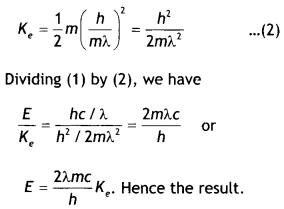Numerical Problems:

Question 1.
What is the de Brogue wavelength associated with an electron, accelerated through a potential difference of 100 volts? (NCERT)
Accelerating potential V = 100 V. The de-Broglie wavelength λ is
λ = $$\frac{1.227}{\sqrt{V}}$$nm = $$\frac{1.227}{\sqrt{100}}$$ = 0.123 nm
The de-Broglie wavelength associated with an electron in this case is of the order of X-ray wavetengths.

Question 2.
In an experiment on the photoelectric effect, the slope of the cut-off voltage versus frequency of incident light is found to be 4.12 × 10-15 V s. Calculate the value of Planck’s constant. (NCERT)
Given slope = 4.12 × 10-15 V s, h = 1

The slope of the voltage versus frequency graph gives the value of h/e, therefore we have
h = Slope × e = 4.12 × 10-15 × 1.6 × 10-19
Or
h = 6.59 × 10-34 J s

Question 3.
The threshold frequency for a certain metal is 3.3 × 1014 Hz. If the light of frequency 8.2 × 1014 Hz is incident on the metal, predict the cut-off voltage for the photoelectric emission. (NCERT)
Given vo = 3.3 × 1014 Hz, v = 8.2 × 1014 Hz, Vo = ?

Using the relation h (v – vo) = eV0 we have h(v – vo)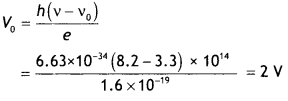Question 4.
The work function for a certain metal is 4.2 eV. Will this metal give photoelectric emission for incident radiation of wavelength 330 nm? (NCERT)
Given ωo = 4.2 eV
The energy possessed by the incident radiation of wavelength 330 nm is
E = $$\frac{h c}{\lambda}=\frac{6.63 \times 10^{-34} \times 3 \times 10^{8}}{330 \times 10^{-9} \times 1.6 \times 10^{-19}}$$ = 3.8 eV

Since this energy is less than the work function of the metal, no photoelectric emission will take place.

Question 5.
Light of frequency 7.21 × 1014 Hz is incident on a metal surface. Electrons with a maximum speed of 6.0 × 10 m s-1 are ejected from the surface. What is the threshold frequency for the photoemission of electrons? (NCERT)
Given v = 7.21 × 1014 Hz, v = 6.0 × 10 m s-1, vo = ?
Using the relation h (v – vo) = $$\frac{1}{2}$$mv² We have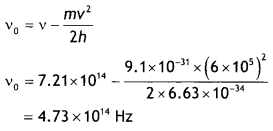Question 6.
Calculate the (a) momentum and (b) de Broglie wavelength of the electrons accelerated through a potential difference of 56 V. (NCERT)
Given V = 56 V, p = ?, λ = ?
Using the relation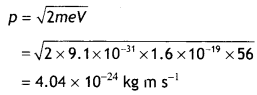The de-Broglie wavelength is given by
λ = $$\frac{h}{p}=\frac{6.63 \times 10^{-34}}{4.04 \times 10^{-24}}$$ = 0.164 nm

Question 7.
A particle is moving three times as fast as an electron. The ratio of the de-Broglie wavelength of the particle to that of the electron is 1.813 × 10-4. Calculate the particle’s mass and identify the particle. (NCERT)
Givenv = 3ve, λ/λe = 1.813 × 10-4
We know that λ = $$\frac{h}{p}=\frac{h}{m v}$$

therefore we have
m = me$$\frac{\lambda_{e} v_{e}}{\lambda v}=\frac{9.11 \times 10^{-31}}{1.813 \times 10^{-4} \times 3}$$

Solving we have m = 1.675 × 10-27 kg
This is the mass of a proton or a neutron.

Question 8.
Using the graph shown in the figure for stopping potential v/s incident frequency of photons, calculate Planck’s constant. (CBSE Delhi 2015C)By Einstein’s equation we have eVo = h(v – vo)

Therefore slope of the graph is $$\frac{V_{0}}{v}=\frac{h}{e}$$. Now slope of graph is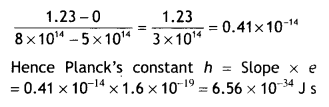Question 9.
If the light of wavelength 412.5 nm is incident on each of the metals given below, which ones will show photoelectric emission and why? (CBSE AI Delhi 2018)

 Metal Work Function (eV) Na 1.92 K 2.15 Ca 3.20 Mo 4.17

The energy of a photon of the given light is,E = 3.01 eV. Thus, metals having a work function less than the energy of a photon of falling light will show the photoelectric effect So, Na and K will show the photoelectric effect.

Question 10.
Two metals A and B have work functions 2 eV and 5 eV respectively. Which metal will emit electrons, when irradiated with light of wavelength 400 nm and why?
The energy possessed by radiation of wavelength 400 nm is E = hv = hc/λ or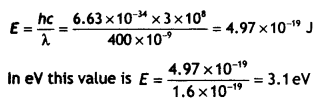Since this energy is more than the work function of metal A, metal A will emit electrons.

Question 11.
Calculate the maximum kinetic energy of electrons emitted from a photosensitive surface of work function 3.2 eV, for the incident radiation of wavelength 300 nm.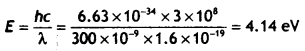Therefore the kinetic energy of the emitted photoelectrons is
K.Emax = hv – hvo = 4.14 – 3.2 = 0.94 ev

Question 12.
The work function, for a given photosensitive surface, equals 2.5 eV. When the light of frequency y falls on this surface, the emitted photoelectron is completely stopped by applying a retarding potential of 4.1 V. What is the value of y?Question 13.
A nucleus of mass M Initially at rest splits into two fragments of masses M’/3 and 2M’/3 (M > M’). Find the ratio of de-Broglie wavelengths of the two fragments.
By the principle of conservation of momentumThe ratio of de-Broglie wavelength is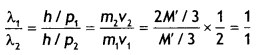Question 14.
An electron microscope uses electrons accelerated by a voltage of 50 kV. Determine the de-Broglie wavelength associated with the electrons. Taking other factors, such as numerical aperture etc. to be the same, how does the resolving power of an electron microscope compare with that of an optical microscope which uses yellow light? (CBSE AI 2014)
Given V = 50 kV, λ = ?
Using the expression λ = $$\frac{1.227}{\sqrt{V}}$$ nm we have
λ = $$\frac{1.227}{\sqrt{50 \times 10^{3}}}$$ nm = 5.48 × 10-12 m

Wavelength of yellow light λy = 5.9 × 10-7 m

Now RP ∝ $$\frac{1}{λ}$$

Therefore we have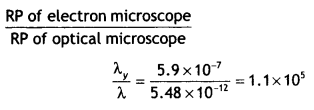Question 15.
A proton and a deuteron are accelerated through the same accelerating potential. Which one of the two has
(a) the greater value of de-Broghe wavelength associated with it and
(a) The de-Broglie wavelength is given by the expression λ = $$\frac{h}{\sqrt{2 m q V}}$$. Since potential is same, we have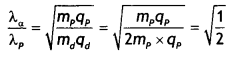Or
λp = $$\sqrt{2} \lambda_{a}$$

Thus proton has a greater value of de-Broglie wavelength.

(b) less momentum? Give reasons to justify your answer. (CBSE Delhi 2014)
Now p = h/λ.

As the wavelength of a proton is more than that of a deuteron, the momentum of a proton is lesser than that of a deuteron. Hence, the momentum of the proton is less.

Question 16.
(a) Monochromatic light of frequency 6.0 × 1014 Hz is produced by a laser. The power emitted is 2.0 × 10-3 W. Estimate the number of photons emitted per second on an average by the source.
Each photon has energy
E = hv = (6.63 × 10-34) × (6.0 × 1014)
= 3.98 × 10-19 J

If N is the number of photons emitted by the source per second, the power P transmitted in the beam equals N times the energy per photon E so that P = NE, then
N = $$\frac{P}{E}=\frac{2.0 \times 10^{-3}}{3.98 \times 10^{-19}}$$
= 5.0 × 1015 photons per second.

(b) Draw a plot showing the variation of photoelectric current versus the intensity of incident radiation on a given photosensitive surface. (CBSE Delhi 2014)
With the increase of intensity of radiations, photocurrent increase.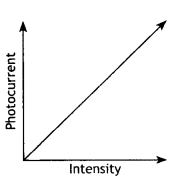Question 17.
An electron is revolving around the nucleus with a constant speed of 2.2 × 108 m s-1. Find the de Broglie wavelength associated with it. (CBSE AI 2014C)
Given v= 2.2 × 108 m s-1, λ = ?
Using the expression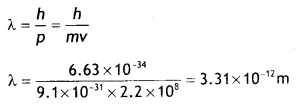Question 18.
Given the ground state energy E0 = – 13.6eV and Bohr radius αo = 0.53 Å. Find out how the de Broglie wavelength associated with the electron orbiting in the ground state would change when it jumps into the first excited state. (CBSE AI 2015)
The de-Broglie wavelength is given by 2πrn = nλ

In ground state, n = 1 and r0 = 0.53 Å, therefore λo = 2 × 3.14 × 0.53 = 3.33 Å
In first excited state, n = 2 and
r1 = 4 × 0.53 Å = 2.12 Å,

therefore λ1 = (2 × 3.14 × 2.12)/2 = 6.66 Å
Therefore λ1 – λo = 6.66 – 3.33 = 3.33 Å

In other words, the de-Broglie wavelength becomes double.

Question 19.
A proton and an a-particle have the same de-Broglie wavelength. Determine the ratio of (i) their accelerating potentials and (ii) their speeds. (CBSE Delhi 2015)
(i) The de Broglie wavelength is given by λ = $$\frac{h}{\sqrt{2 m q V}}$$. Since the de-Broglie waveLength is the same (or proton and alpha particle, we have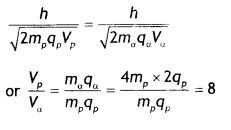(ii) The de-Broglie wavelength is given by λ = $$\frac{h}{mv}$$. Since the de-Broglie wavelength is the same (or proton and alpha particle, we have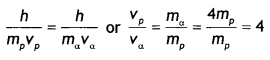Question 20.
The work function (ωo), of a metal X, equals 3 × 10-19 J. Calculate the number (N) of photons, of light of wavelength 26.52 nm, whose total energy equals W. (CBSE Delhi 2016C)
Given ωoo = 3 × 10-19 J, N = ?, λ = 26.52 nm = 26.52 × 10-9 m
The energy possessed by one photonTherefore the number of photons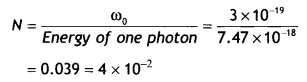Question 21.
The KE of a beam of electrons accelerated through a potential V, equals the energy of a photon of wavelength 5460 nm. Find the de Broglie wavelength associated with this beam of electrons. (CBSE AI 2016C)
The de-Broglie wavelength is given byQuestion 22.
Calculate the kinetic energy of an electron having de Broglie wavelength of 1 A. (CBSE AI 2017C)
Using the relation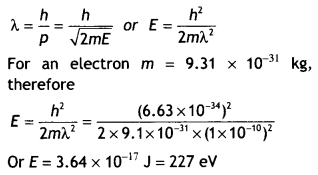Question 23.
Find the frequency of light that ejects electrons from a metal surface, fully stopped by a retarding potential of 3.3 V. If photoelectric emission begins in this metal at a frequency of 8 × 1014 Hz, calculate the work function (in eV) for this metal. (CBSE AI 2018C)
The work function is given by ω0 = hv0Question 24.
Monochromatic light of frequency 6.0 × 1014 Hz is produced by a laser. The power emitted is 2.0 × 10-3 W. Calculate the (i) energy of a photon in the light beam and
Each photon has an energy
E = hv = (6.63 × 10-34)(6.0 × 1014)
= 3.98 × 10-19 J

(ii) a number of photons emitted on an average by the source. (CBSE AI 2018C)
If N is the number of photons emitted by the source per second, the power P transmitted in the beam equals N times the energy per photon E so that P = NE. Then
N = $$\frac{P}{E}=\frac{2.0 \times 10^{-3}}{3.98 \times 10^{-19}}$$
= 5.0 × 1015 photons per second.

Question 25.
The following table gives the values of work function for a few photosensitive metals.

 S.No. Metal Work Function (eV) 1. Na 1.92 2. K 2.15 3. Ca 3.20 4. Mo 4.17

If each of these metals is exposed to radiations of wavelength 300 nm, which of them will not emit photoelectrons and why?
The energy of the radiation is
E = $$\frac{h c}{\lambda}=\frac{6.63 \times 10^{-34} \times 3 \times 10^{8}}{300 \times 10^{-9} \times 1.6 \times 10^{-19}}$$ = 4.14 eV

This energy is greater than the work functions of Na and K and lesser than the work function of Mo. Hence Mo will not emit photoelectrons.

Question 26.
The work function of caesium is 2.14 eV. Find
(i) the threshold frequency for caesium, and
For the cut-off or threshold frequency the energy hv0 of the incident radiation must be equal to work function Φ0 so that
v0 = $$\frac{\phi_{0}}{h}=\frac{2.14 \times 1.6 \times 10^{-19}}{6.63 \times 10^{-34}}$$
= 5.16 × 1014 Hz
Thus, for frequencies less than this threshold frequency, no photoelectrons are ejected.

(ii) the wavelength of the incident light if the photocurrent is brought to zero by a stopping potential of 0.60 V. (NCERT)
Photocurrent reduces to zero when the maximum kinetic energy of the emitted photoelectrons equals the potential energy eV0 by the retarding potential V0.
Einstein’s Photoelectric equation is eV0 = hv – ω0 = $$\frac{hc}{λ}$$ – ω0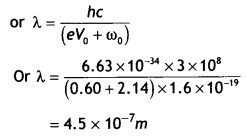Question 27.
An electron, an a-particle, and a proton have the same kinetic energy. Which of these particles has the shortest de-Broglie wavelength? (NCERT)
For a particle, de-Broglie wavelength λ = h/p
Kinetic energy, K = p2/2m.
Then, λ = $$\frac{h}{\sqrt{2 m K}}$$

For the same kinetic energy K, the de-Broglie wavelength associated with the particle is inversely proportional to the square root of their masses. A proton (11H) is 1836 times massive than an electron and a particle (24He) four times that of a proton. Hence, a-particle has the shortest de-Broglie wavelength.

Question 28.
A particle is moving three times as fast as an electron. The ratio of the de Broglie wavelength of the particle to that of the electron is 1.813 × 10-4. Calculate the particle’s mass and identify the particle. (NCERT)
the de-Broglie wavelength of a moving particle, having mass m and velocity v
λ = $$\frac{h}{p}=\frac{h}{m v}$$

Mass, m = h/λv
For an electron, mass me = h/λe ve
Now, we have v/ve = 3 and λ/λe = 1.813 × 10-4

Then the mass of the particleThus, the particle with this mass could be a proton or a neutron.

Question 29.
What are the (a) momentum, (b) speed, and (c) de Brogue wavelength of an electron with the kinetic energy of 120 eV? (NCERT)
Given E = 120 eV, p =?, v =?, λ =?
Using the relation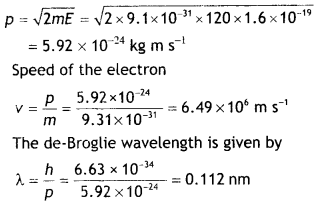Question 30.
Consider a metal exposed to the light of wavelength 600 nm. The maximum energy of the electron doubles when the light of wavelength 400 nm is used. Find the work function in eV. (NCERT Exemplar)
Given λ1 = 600 nm, E1 = E, λ2 = 400 nm,
E2 = 2E, Φ =?
Now Emax = hv — Φ

According to the question (hc = 1230 eVnm)Question 31.
A student performs an experiment on the photoelectric effect, using two materials A and B. A plot of vis given In the figure.
(a) Which material A or B has a higher work function?
(b) Given the electric charge of an electron = 1.6 × 10-19 C, find the value of h obtained from the experiment for both A and B. Comment on whether it is consistent with Einstein’s theory. (NCERT Exemplar)